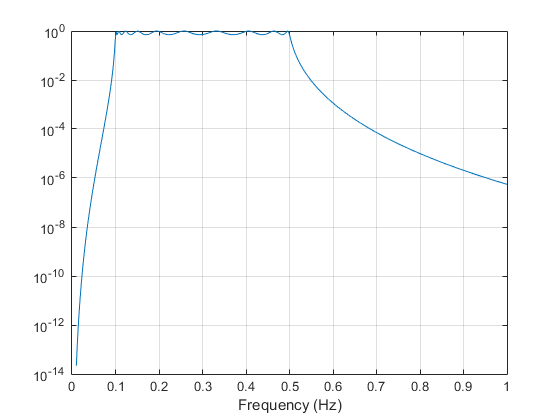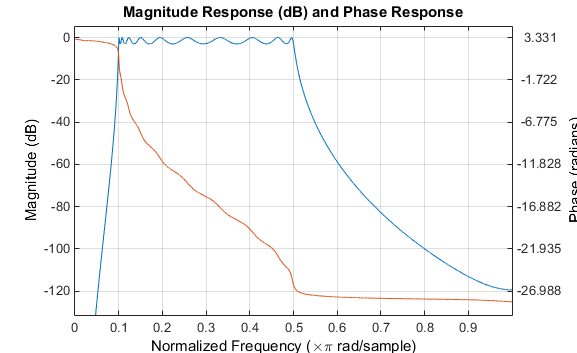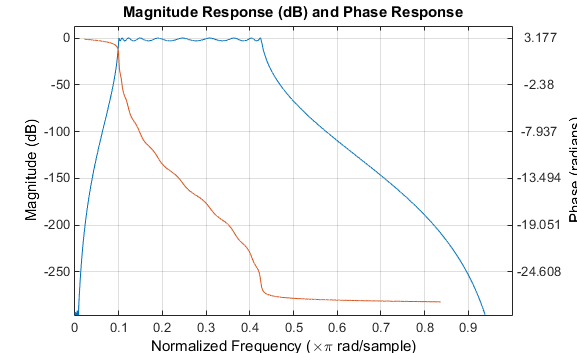## Special Topics in IIR Filter Design

### Classic IIR Filter Design

The classic IIR filter design technique includes the following steps.

1. Find an analog lowpass filter with cutoff frequency of 1 and translate this prototype filter to the specified band configuration

2. Transform the filter to the digital domain.

3. Discretize the filter.

The toolbox provides functions for each of these steps.

Available functions

Analog lowpass prototype

Frequency transformation

Discretization

Alternatively, the `butter`, `cheby1`, `cheb2ord`, `ellip`, and `besself` functions perform all steps of the filter design and the `buttord`, `cheb1ord`, `cheb2ord`, and `ellipord` functions provide minimum order computation for IIR filters. These functions are sufficient for many design problems, and the lower level functions are generally not needed. But if you do have an application where you need to transform the band edges of an analog filter, or discretize a rational transfer function, this section describes the tools with which to do so.

### Analog Prototype Design

This toolbox provides a number of functions to create lowpass analog prototype filters with cutoff frequency of 1, the first step in the classical approach to IIR filter design.

The table below summarizes the analog prototype design functions for each supported filter type; plots for each type are shown in IIR Filter Design.

Filter Type

Analog Prototype Function

Bessel

`[z,p,k] = besselap(n)`

Butterworth

`[z,p,k] = buttap(n)`

Chebyshev Type I

`[z,p,k] = cheb1ap(n,Rp)`

Chebyshev Type II

`[z,p,k] = cheb2ap(n,Rs)`

Elliptic

`[z,p,k] = ellipap(n,Rp,Rs)`

### Frequency Transformation

The second step in the analog prototyping design technique is the frequency transformation of a lowpass prototype. The toolbox provides a set of functions to transform analog lowpass prototypes (with cutoff frequency of 1 rad/s) into bandpass, highpass, bandstop, and lowpass filters with the specified cutoff frequency.

Frequency Transformation

Transformation Function

Lowpass to lowpass

`${s}^{\prime }=s/{\omega }_{0}$`

```[numt,dent]   = lp2lp (num,den,Wo)```

```[At,Bt,Ct,Dt] = lp2lp (A,B,C,D,Wo)```

Lowpass to highpass

`${s}^{\prime }=\frac{{\omega }_{0}}{s}$`

```[numt,dent]   = lp2hp (num,den,Wo)```

```[At,Bt,Ct,Dt] = lp2hp (A,B,C,D,Wo)```

Lowpass to bandpass

`${s}^{\prime }=\frac{{\omega }_{0}}{{B}_{\omega }}\frac{{\left(s/{\omega }_{0}\right)}^{2}+1}{s/{\omega }_{0}}$`

```[numt,dent]   = lp2bp (num,den,Wo,Bw)```

```[At,Bt,Ct,Dt] = lp2bp (A,B,C,D,Wo,Bw)```

Lowpass to bandstop

`${s}^{\prime }=\frac{{B}_{\omega }}{{\omega }_{0}}\frac{s/{\omega }_{0}}{{\left(s/{\omega }_{0}\right)}^{2}+1}$`

```[numt,dent]   = lp2bs (num,den,Wo,Bw)```

```[At,Bt,Ct,Dt] = lp2bs( A,B,C,D,Wo,Bw)```

As shown, all of the frequency transformation functions can accept two linear system models: transfer function and state-space form. For the bandpass and bandstop cases

`${\omega }_{0}=\sqrt{{\omega }_{1}{\omega }_{2}}$`

and

`${B}_{\omega }={\omega }_{2}-{\omega }_{1}$`

where ω1 is the lower band edge and ω2 is the upper band edge.

The frequency transformation functions perform frequency variable substitution. In the case of `lp2bp` and `lp2bs`, this is a second-order substitution, so the output filter is twice the order of the input. For `lp2lp` and `lp2hp`, the output filter is the same order as the input.

To begin designing an order 10 bandpass Chebyshev Type I filter with a value of 3 dB for passband ripple, enter

```[z,p,k] = cheb1ap(10,3); ```

Outputs `z`, `p`, and `k` contain the zeros, poles, and gain of a lowpass analog filter with cutoff frequency Ωc equal to 1 rad/s. Use the function to transform this lowpass prototype to a bandpass analog filter with band edges Ω1 = π/5 and Ω2 = π. First, convert the filter to state-space form so the `lp2bp` function can accept it:

`[A,B,C,D] = zp2ss(z,p,k); % Convert to state-space form.`

Now, find the bandwidth and center frequency, and call `lp2bp`:

```u1 = 0.1*2*pi; u2 = 0.5*2*pi; % In radians per second Bw = u2-u1; Wo = sqrt(u1*u2); [At,Bt,Ct,Dt] = lp2bp(A,B,C,D,Wo,Bw);```

Finally, calculate the frequency response and plot its magnitude:

```[b,a] = ss2tf(At,Bt,Ct,Dt); % Convert to TF form w = linspace(0.01,1,500)*2*pi; % Generate frequency vector h = freqs(b,a,w); % Compute frequency response semilogy(w/2/pi,abs(h)) % Plot log magnitude vs. freq xlabel('Frequency (Hz)') grid```### Filter Discretization

The third step in the analog prototyping technique is the transformation of the filter to the discrete-time domain. The toolbox provides two methods for this: the impulse invariant and bilinear transformations. The filter design functions `butter`, `cheby1`, `cheby2`, and `ellip` use the bilinear transformation for discretization in this step.

Analog to Digital Transformation

Transformation Function

Impulse invariance

```[numd,dend] = impinvar (num,den,fs)```

Bilinear transform

```[zd,pd,kd] = bilinear (z,p,k,fs,Fp)```

```[numd,dend] = bilinear (num,den,fs,Fp)```

```[Ad,Bd,Cd,Dd] = bilinear (At,Bt,Ct,Dt,fs,Fp)```

#### Impulse Invariance

The toolbox function `impinvar` creates a digital filter whose impulse response is the samples of the continuous impulse response of an analog filter. This function works only on filters in transfer function form. For best results, the analog filter should have negligible frequency content above half the sampling frequency, because such high-frequency content is aliased into lower bands upon sampling. Impulse invariance works for some lowpass and bandpass filters, but is not appropriate for highpass and bandstop filters.

Design a Chebyshev Type I filter and plot its frequency and phase response using FVTool:

```[bz,az] = impinvar(b,a,2); fvtool(bz,az) ```

Click the toolbar button.Impulse invariance retains the cutoff frequencies of 0.1 Hz and 0.5 Hz.

#### Bilinear Transformation

The bilinear transformation is a nonlinear mapping of the continuous domain to the discrete domain; it maps the s-plane into the z-plane by

`$H\left(z\right)=H\left(s\right)|{}_{s=k\frac{z-1}{z+1}}$`

Bilinear transformation maps the jΩ-axis of the continuous domain to the unit circle of the discrete domain according to

`$\omega =2{\mathrm{tan}}^{-1}\left(\frac{\Omega }{k}\right)$`

The toolbox function `bilinear` implements this operation, where the frequency warping constant k is equal to twice the sampling frequency (`2*fs`) by default, and equal to $2\pi {f}_{p}/\mathrm{tan}\left(\pi {f}_{p}/{f}_{s}\right)$if you give `bilinear` a trailing argument that represents a “match” frequency `Fp`. If a match frequency `Fp` (in hertz) is present, `bilinear` maps the frequency Ω = 2πfp (in rad/s) to the same frequency in the discrete domain, normalized to the sampling rate: ω = 2πfp/fs (in rad/sample).

The `bilinear` function can perform this transformation on three different linear system representations: zero-pole-gain, transfer function, and state-space form. Try calling `bilinear` with the state-space matrices that describe the Chebyshev Type I filter from the previous section, using a sampling frequency of 2 Hz, and retaining the lower band edge of 0.1 Hz:

```[Ad,Bd,Cd,Dd] = bilinear(At,Bt,Ct,Dt,2,0.1); ```

The frequency response of the resulting digital filter is

```[bz,az] = ss2tf(Ad,Bd,Cd,Dd); % Convert to TF fvtool(bz,az) ```

Click the toolbar button.The lower band edge is at 0.1 Hz as expected. Notice, however, that the upper band edge is slightly less than 0.5 Hz, although in the analog domain it was exactly 0.5 Hz. This illustrates the nonlinear nature of the bilinear transformation. To counteract this nonlinearity, it is necessary to create analog domain filters with “prewarped” band edges, which map to the correct locations upon bilinear transformation. Here the prewarped frequencies `u1` and `u2` generate `Bw` and `Wo` for the `lp2bp` function:

```fs = 2; % Sampling frequency (hertz) u1 = 2*fs*tan(0.1*(2*pi/fs)/2); % Lower band edge (rad/s) u2 = 2*fs*tan(0.5*(2*pi/fs)/2); % Upper band edge (rad/s) Bw = u2 - u1; % Bandwidth Wo = sqrt(u1*u2); % Center frequency [At,Bt,Ct,Dt] = lp2bp(A,B,C,D,Wo,Bw); ```

A digital bandpass filter with correct band edges 0.1 and 0.5 times the Nyquist frequency is

```[Ad,Bd,Cd,Dd] = bilinear(At,Bt,Ct,Dt,fs); ```

The example bandpass filters from the last two sections could also be created in one statement using the complete IIR design function `cheby1`. For instance, an analog version of the example Chebyshev filter is

```[b,a] = cheby1(5,3,[0.1 0.5]*2*pi,'s'); ```

Note that the band edges are in rad/s for analog filters, whereas for the digital case, frequency is normalized:

```[bz,az] = cheby1(5,3,[0.1 0.5]); ```

All of the complete design functions call `bilinear` internally. They prewarp the band edges as needed to obtain the correct digital filter.